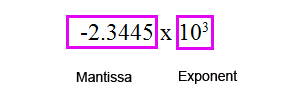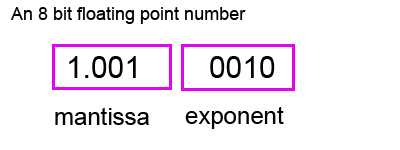# 6. Floating point

There is an efficient method of representing a 'real number' in a binary form.

It is called floating point because effectively the location of the decimal/binary point moves around.

Going back to decimal numbers for a moment, you can represent a number in scientific notation as followsThe first part is called the 'mantissa'. The mantissa also holds the sign of the number. The second part is called the 'exponent' and it defines where the decimal point needs to be if the number is shown in its standard decimal form. In this case it indicates that this number is to be multiplied by a thousand and so the decimal point moves 3 locations to the right, like this

-2344.5

Binary floating point uses the same idea. A binary floating point number is in two parts. The Mantissa and the Exponent.Both the mantissa and the exponent is in twos complement format. So if there is a 1 present in the leftmost bit of the mantissa, then this is a negative binary number.

The exponent part is indicating where the decimal point needs to be.

Let's break down this particular number and see what it represents.

1.001 mantissa. The most significant bit is a 1 and we know it is in two's complement so this must be a negative number.

0010 exponent. This indicates how many places the the decimal point needs to move. If it is a positive exponent, then you move the decimal point to the right. If it was a negative exponent, then you would slide the decimal point to the left.

The exponent is 0010, so this is a positive 2. Slide the decimal point of the mantissa 2 positions to the right.

So the full binary number is

1.001 becomes 100.1

It is in two complement and it is a negative number so flip the bits to the left of the last 1

011.1

The number is -3.5 decimal

`     `